# scale

Scale geometry

Since R2020a

## Syntax

``h = scale(g,s)``
``h = scale(g,s,refpoint)``

## Description

example

````h = scale(g,s)` scales the geometry `g` by the factor `s` with respect to the origin.```

example

````h = scale(g,s,refpoint)` scales the geometry with respect to the reference point `refpoint`.```

## Examples

collapse all

Scale a 2-D geometry along the x- and y-axis and ensure consistency with the mesh.

Create a model.

`model = createpde;`

Import and plot a geometry.

```g = importGeometry(model,"PlateHolePlanar.stl"); pdegplot(model)```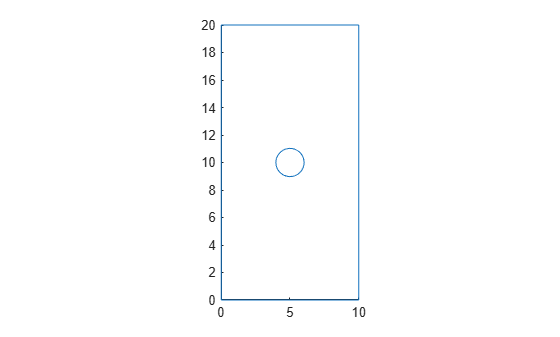Mesh the geometry and plot the mesh.

```generateMesh(model); figure pdemesh(model)```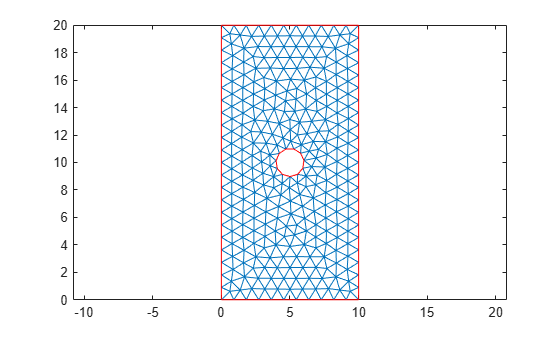Scale the geometry by a factor of 10 along the x-axis.

`scale(g,[10 1])`
```ans = DiscreteGeometry with properties: NumCells: 0 NumFaces: 1 NumEdges: 5 NumVertices: 5 Vertices: [5x3 double] ```

Plot the geometry.

```figure pdegplot(model)```Plot the geometry and mesh. The `scale` function modifies a geometry, but it does not modify a mesh.

```figure pdegplot(model) hold on pdemesh(model)```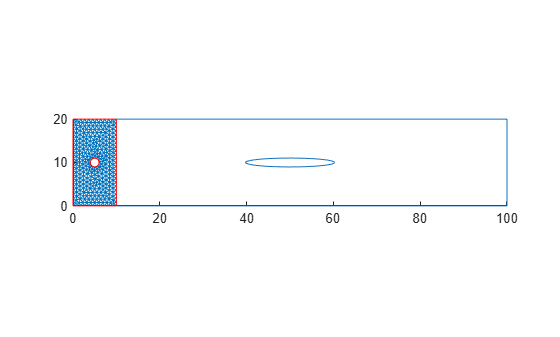After modifying the geometry, always regenerate the mesh.

```generateMesh(model); figure pdegplot(model) hold on pdemesh(model)```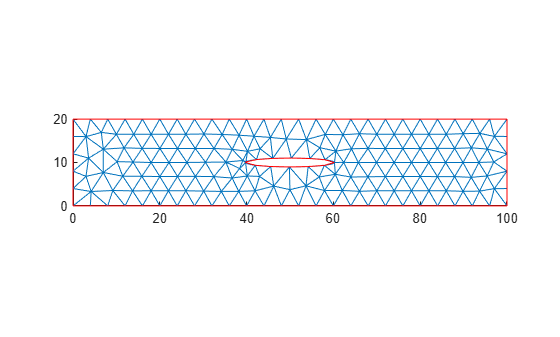Reflect the geometry across the x-axis and regenerate the mesh.

```scale(g,[1 -1]); generateMesh(model);```

Plot the resulting geometry and mesh.

```figure subplot(2,1,1) pdegplot(model) subplot(2,1,2) pdemesh(model)```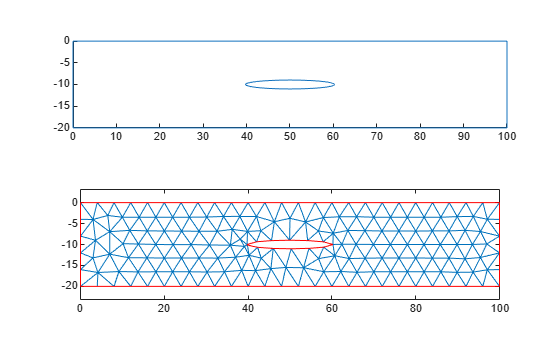Enlarge a geometry: first uniformly in all directions, and then using different scaling factors along different axes.

Create and plot a geometry.

```g = multicuboid(1,1,1); pdegplot(g,"VertexLabels","on","FaceAlpha",0.5)```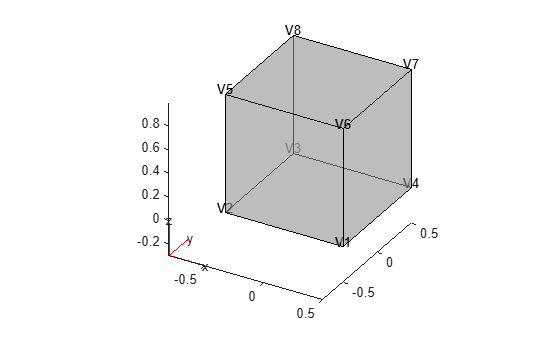Scale the geometry by a factor of 2 uniformly along all coordinate axes. Plot the result.

```scale(g,2); pdegplot(g,"VertexLabels","on","FaceAlpha",0.5)```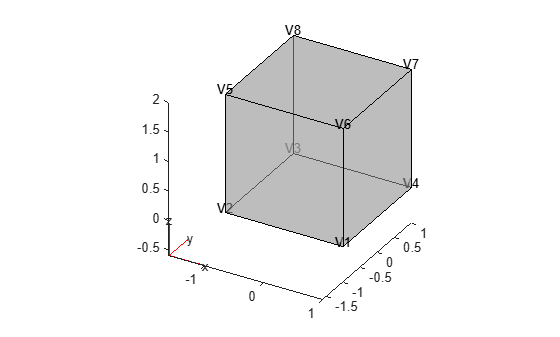Now scale by factors of 2, 3, and 4 along the x-, y-, and z-axes, respectively. Plot the result.

```scale(g,[2 3 4]); pdegplot(g,"VertexLabels","on","FaceAlpha",0.5)```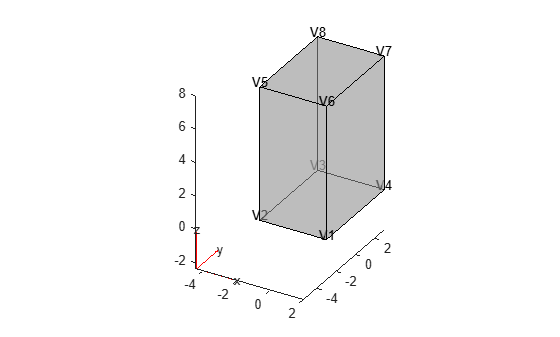Flip the geometry upside down by scaling it with the factor `-1` and using the bottom front corner (vertex 1) as a reference point.

```scale(g,[1 1 -1], [2 -3 0]); pdegplot(g,"VertexLabels","on","FaceAlpha",0.5)```## Input Arguments

collapse all

Geometry, specified as an `fegeometry` object, a `DiscreteGeometry` object, or an `AnalyticGeometry` object.

Scaling factor, specified as a real number or vector of two or three real numbers. Use one value for uniform scaling in all directions. Use a vector of two or three elements to specify different scaling factors along the x-, y-, and, for a 3-D geometry, z-axes.

Reference point for scaling specified as a vector of two or three real numbers for a 2-D and 3-D geometry, respectively

## Output Arguments

collapse all

Resulting geometry, returned as an `fegeometry` object or a handle.

• If the original geometry `g` is an `fegeometry` object, then `h` is a new `fegeometry` object representing the modified geometry. The original geometry `g` remains unchanged.

• If the original geometry `g` is a `DiscreteGeometry` object, then `h` is a handle to the modified `DiscreteGeometry` object `g`.

• If `g` is an `AnalyticGeometry` object, then `h` is a handle to a new `DiscreteGeometry` object. The original geometry `g` remains unchanged.

## Tips

• After modifying a geometry, regenerate the mesh to ensure a proper mesh association with the new geometry.

• If the scaling factor is negative, then the coordinates will flip their signs. The scaling factor of `-1` mirrors the existing geometry if the reference point is the origin.

• If `g` is an `fegeometry` or `AnalyticGeometry` object, and you want to replace it with the modified geometry, assign the output to the original geometry, for example, ```g = scale(g,20)```.

## Version History

Introduced in R2020a

expand all